Register for Maths, Science, English, Reasoning Olympiad Exams Register here | Book Free Demo for Live Olympiad Classes here| Check Olympiad Exam Dates here | Buy Practice Papers for IMO, IOM, HEO, IOEL etc here | Login here to participate in all India free mock test on every Saturday

Motion and Measurement of Distance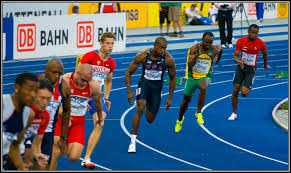Long time ago, there were no means of transport. People had to walk on rugged paths with heavy loads on their back. But since the invention of the wheel, Motion through non-living things was made possible and ever since there has been an ever-increasing development in transportation means. Motion and measurement of distance are important aspects of transportation.

Measuring Instruments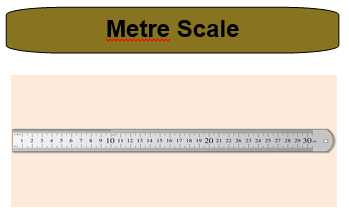Least count= 0.1cm=1mm

The instruments which are used to measure small items to the accuracy of 1      mm.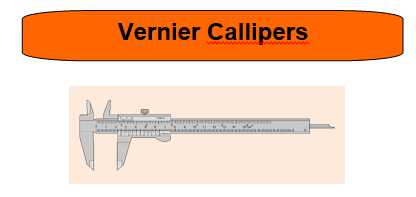Least Count: 0.1mm

This instrument is used to measure objects up to the accuracy of 0.1mm.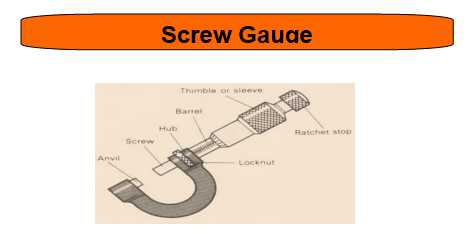Least Count: 0.1 mm

This instrument is used to measure an object up to the accuracy of 0.1mm.

Units of Measurement

In ancient times, the people of the Indus valley civilization used very good measurements of length because we see can evidence in excavations of perfectly geometrical constructions. A cubit is the length from the elbow to the finger tips was used in ancient Egypt and was also accepted as a unit of length in other parts of the world. People also used foot as a unit of length in different parts of the world. The length of the foot used varied slightly from region to region depending from person to person. People will measure a yard of cloth by the distance between the end of the outstretched arm and their chin. The Romans used to measure with their pace or steps.

Even today, we see flower sellers using their forearm as a unit of length for garlands in many towns of India. Many such body parts continue to be in use as unit of length, when convenient. However, everyone's body parts could be of slightly different sizes. This must have caused confusion in measurement. In 1790, the French created a standard unit of measurement called the metric system. For the sake of maintaining standards, scientists all over the world have accepted a set of standard units of measurement. The system of units now used is known as the International System of Units (SI units).

The SI unit of length is a metre. A metre scale is shown.  Also shown is the 15 cm scale in your geometry box. Each metre (m) is divided into 100 equal divisions, called centimetre (cm). Each centimetre has ten equal divisions, called millimetre (mm). Thus, 1 m = 100 cm 1 cm = 10 mm. For measuring large distances, metre is not a convenient unit. We define a larger unit of length. It is called kilometre (km). 1 km = 1000 m. Now, we can repeat all our measurement activities using a standard scale and measure in SI units.

1. First, place the scale in contact with the object along its length.
2. In some scales, the ends is broken. You should be able to see the zero mark. We should avoid taking measurements with zero mark of the scale. You can use any full mark of the scale. Then subtract the reading of this mark from the reading at the other end.
3. Correct position of the eye is important for taking measurement. Your eye be exactly in front of the point where the measurement is to be taken as shown. Note that from position 'B', the reading is 7.5 cm. From positions, the readings may be different.

Types of Motion

• Rectilinear Motion: These motion continue in a straight line.
• Circular Motion: This motion changes its direction at every single point.

Recap

• Least count of a metre scale is 1mm.
• Least count of a vernier scale is 0.1mm.
• Least count of screw gauge is 0.1 mm.
• There are two types of motion: Rectilinear and circular motion.
• Rectilinear motion is motion in a straight line.
• Circular motion changes its direction at all points.Quiz for Motion and Measurement of Distance

 Q.1 The area under velocity- time graph gives the a) Velocity of the body b) Displacement of the body c) Acceleration of the body d) Distance of the body
 Q.2 Which of the following velocity- time graph represents uniform acceleration? a)b)c)d)Q.3 Which of the following is a false statement? a) A body can have a constant speed and still have varying velocity b) The direction of the velocity of a body can change when its acceleration is constant. c) A body can have zero velocity and still be accelerated. d) A body can have a constant velocity and still have a varying speed.
 Q.4 The following graph represents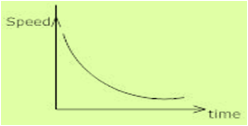a) Constant velocity b) Constant retardation c) Non- uniform acceleration d) Constant acceleration
 Q.5 In a circular track (distance 400 m) an athlete runs 1/4 the of the ground. So what would be the displacement?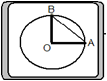a) 80 m b) 90 m c) 92 m d) 95 m
 Q.6 A train travels 40 km at a uniform speed of 30 km/hr. Its average speed after travelling another 40 km is 45 km/hr for the whole journey. Find it's speed in the second half of the journey? a) 90 km/h b) 60 km/h c) 120 km/h d) 80 km/hr
 Q.7 A car moves with a speed of 30km/h for half an hour; 25km/h for 1 hr and 40km/hr for 2 hrs. Find average speed? a) 60 km/h b) 120 km/h c) 90 km/hr d) 80 km/hr
 Q.8 A 150 metres long train crosses a man walking at the speed of 6km/h in the opposite direction in 6 seconds. Find the speed of the train in km/h? a) 60 km/h b) 80 km/h c) 84 km/h d) 90 km/h
 Q.9 A train acquire velocity of 80 km/h in just half an hour after start. Find its acceleration? a) 0.11 m/s2 b) 0.12 m/s2 c) 0.1 m/s2 d) 0.15 m/s2
 Q.10 Car is travelling along the road at 8m/s. It accelerates at 1m/s2 for a distance of 18m. How fast is it travelling? a) 12 m/s b) 15 m/s c) 10 m/s d) 8 m/s